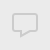## 统计基础

#### dataframe的index索引是否可以重复

df = pd.DataFrame({'A': [1, 2, 3, 4, 5], 'B': [10, 20, 30, 40, 50]})
df2 = pd.DataFrame({'A': , 'B': })
print 'df\n', df
print 'df2\n', df2输出如下：
df1

df2

result = pd.concat(df_x)
print 'first result\n', result

查看全部

```    df = pd.DataFrame({'A': [1, 2, 3, 4, 5], 'B': [10, 20, 30, 40, 50]})
df2 = pd.DataFrame({'A': , 'B': })
print 'df\n', df
print 'df2\n', df2```

df1

df2

```    df_x = [df, df2]
result = pd.concat(df_x)
print 'first result\n', result```

#### dataframe的index索引是否可以重复

df = pd.DataFrame({'A': [1, 2, 3, 4, 5], 'B': [10, 20, 30, 40, 50]})
df2 = pd.DataFrame({'A': , 'B': })
print 'df\n', df
print 'df2\n', df2输出如下：
df1

df2

result = pd.concat(df_x)
print 'first result\n', result

查看全部

```    df = pd.DataFrame({'A': [1, 2, 3, 4, 5], 'B': [10, 20, 30, 40, 50]})
df2 = pd.DataFrame({'A': , 'B': })
print 'df\n', df
print 'df2\n', df2```

df1

df2

```    df_x = [df, df2]
result = pd.concat(df_x)
print 'first result\n', result```